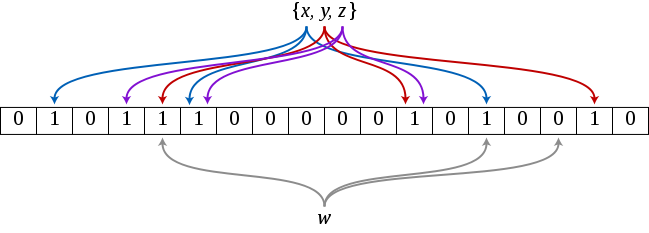## 原理

A Bloom filter is a space-efficient probabilistic data structure, conceived by Burton Howard Bloom in 1970, that is used to test whether an element is a member of a set. False positive matches are possible, but false negatives are not – in other words, a query returns either "possibly in set" or "definitely not in set". Elements can be added to the set, but not removed (though this can be addressed with a "counting" filter); the more elements that are added to the set, the larger the probability of false positives.

## 算法描述• 元素数量 n 越少，生成的 k 个哈希值碰撞的可能就越小，判断也就越准确；
• 数组长度 m 越大，生成的 k 个哈希值碰撞的可能也就越小，判断也就越准确；
• k 个函数选取越准确，生成的 k 个哈希值碰撞的可能越小，判断越准确；

## 误判概率

$$\frac{1}{m}$$

$$1-\frac{1}{m}$$

$$(1-\frac{1}{m})^k$$

$$(1-\frac{1}{m})^{nk}$$

$$1-(1-\frac{1}{m})^{nk}$$

$$P=[1-(1-\frac{1}{m})^{nk}]^k$$

$$\lim_{x \to \infty}(1+x)^{\frac{1}{x}} \approx e$$

$$(1-\frac{1}{m})^{nk}=(1-\frac{1}{m})^{nk \times \frac{-m}{-m}}=((1-\frac{1}{m})^{-m})^{-\frac{nk}{m}} \approx e^{-\frac{nk}{m}}$$

$$P \approx (1-e^{-\frac{nk}{m}})^k$$

## 哈希函数的最佳个数k

$$P \approx (1-e^{-\frac{nk}{m}})^k$$

$$b=e^{\frac{n}{m}}$$

$$P=(1-b^{-k})^k$$

$$\ln P=k \times \ln(1-b^{-k})$$

$$\frac{1}{P} \times P^{'} = \ln(1-b^{-k})+k \times \frac{b^{-k} \times \ln b}{1-b^{-k}}$$

$$k=\frac{m}{n} \times \ln 2$$

[画外音：这个数字熟悉不]

## 布隆过滤器的使用场景

• Google 的分布式数据库 Bigtable 使用布隆过滤器来减少对磁盘的 I/O 次数。当一次查询请求到来时，先在 bloom filter 中查找，若不存在则直接返回，可以提高性能；
• 网络爬虫程序中，需要对抓取到的 URL 进行遍历。若该 URL 之前已经访问过，则可以快速从 bloom filter 中感知到并跳过，否则访问之并加入到 bloom filter 中。当然，出现小概率误判时，don't care it.
• 黑名单过滤。比如在网络请求中，可以将恶意访问的用户 id 或 IP 加入到布隆过滤器中，以阻止这些访问请求，起到防刷的作用。这里布隆过滤器的作用主要是降低黑名单的存储成本。类似的，还可以用于垃圾邮件过滤、垃圾短信过滤、恶意网址过滤等。